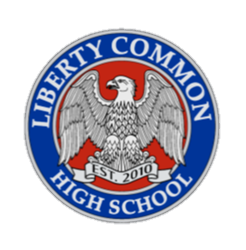T  H  E      B  L  U  E

# P L A N E T

F E B R U A R Y   1 8 T H   I N   B O U L D E R ,   C O L O R A D O

A P R I L   2 0 S T - 2 3 R D   I N   C O R V A L L I S ,   O R E G O N